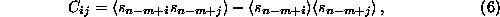Next: Visualizationnon-stationarity Up: Phase space representation Previous: Poincaré sections

## SVD filters

There are at least two reasons to apply an SVD filter to time series data: Either, if one is working with flow data, one can implicitly determine the optimal time delay, or, when deriving a stroboscopic map from synchronously sampled data of a periodically driven system, one might use the redundancy to optimize the signal to noise ratio.

In both applications the mathematics is the same: One constructs the covariance matrix of all data vectors (e.g. in an m-dimensional time delay embedding space),and computes its singular vectors. Then one projects onto the m-dimensional vectors corresponding to the q largest singular values. To work with flow data, q should be at least the correct embedding dimension, and m considerably larger (e.g. m=2q or larger). The result is a vector valued time series, and in  the relation of these components to temporal derivatives on the one hand and to Fourier components on the other hand were discussed. If, in the non-autonomous case, one wants to compress flow data to map data, q=1. In this case, the redundancy of the flow is implicitly used for noise reduction of the map data. The routine svd can be used for both purposes.Next: Visualizationnon-stationarity Up: Phase space representation Previous: Poincaré sections

Thomas Schreiber
Wed Jan 6 15:38:27 CET 1999